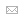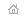українська## The post-war revival of the Institute of Mathematics (1945 - 1959)

In 1945, the Institute of Mathematics was headed again by M. A. Lavrentyev, later - by O. Yu. Ishlinsky (1948-1955) and B. V. Gnedenko (1955-1958).

During this period, the main activities of the Institute were researches on conformal and quasiconformal mappings, spectral operator theory, nonlinear problems of mathematical physics, qualitative theory of differential equations, the development of methods for approximating functions and on the mechanics of a continuous medium.

In 1946, on the initiative of M.A. Lavrentyev, at the institute was created a special laboratory - a comprehensive laboratory for studying the effects of the explosion, which in 1947 was headed by M. M. Sity. The successful work of the laboratory was assisted by the bold idea of M. A. Lavrentyev that the material of the steel shell and armor behaves in extreme conditions as an ideal incompressible liquid. This idea allowed to obtain not only a qualitative picture of the cumulative effect, but also the formulas for the corresponding calculations confirmed by experiments. It was during this period that under the direction of M. A. Lavrentyev was discovered the phenomenon of welding by an explosion that pass ahead for 10 years of the first reports on this subject from the United States.

In 1946, for the development of a variational-geometric method for solving nonlinear problems of the theory of differential equations with partial derivatives that are important for hydro- and aeromechanics M. A. Lavrentyev was awarded the State Prize of the USSR and in 1949 he was awarded for the second time for research in the field of hydromechanics.

In 1945 - 1949 M. M. Bogolyubov had passed to the Institute of Mathematics, continued research on the theory of nonlinear oscillations, which were begun in the 1930's at the Department of Mathematical Physics of the Institute of Construction Mechanics in conjunction with M. M. Krylov, solved complicated the problems of this theory, created the method of averaging, the one-frequency method and the method of integral manifolds and obtained fundamental results in the theory of almost periodic functions and the metric theory of dynamical systems.

In 1947, the scientific results of M. M. Bogolyubov of this period were acknowledged by the State Prize of the USSR.

At the same time, M. M. Bogolyubov performed works devoted to the questions of statistical mechanics of classical and quantum systems, the development of methods for constructing solutions of equations for the functions of distribution and derivation of kinetic equations, and the method of approximate secondary quantization. In 1947, was created a microscopic theory of superfluity. On this basis later was constructed a consistent microscopic theory of superconductivity and superfluidity of nuclear matter.

At the end of 1949, the institute consisted of 7 departments: the theory of functions of a complex variable (the head was M. A. Lavrentyev), algebras and functional analysis (M. G. Krein), asymptotic methods and probability theory (M. M. Bogolyubov), applied mathematics (O. Yu. Ishlinsky), mechanics (Yu. D. Sokolov), Lviv department of probability theory (B. V. Gnedenko), Lviv department of mathematical theory of elasticity (G. M. Savin). Then were created the Laboratory of Computational Mathematics and Engineering (B. V. Gnedenko) and the Laboratory for the Modeling of Higher Nervous Activity (M. M. Amosov).

In his works of this period O. Yu. Ishlinsky conducted extensive researches in the theory of plasticity, friction theory, oscillation theory, general mechanics, instrument making, obtained fundamental results in the theory of gyroscopes and inertial navigation systems.

B. V. Gnedenko developed a study on probability theory, mathematical statistics, mathematical analysis and mathematics history. During his years at the institute, he completed the general theory of summation of random variables, proved local boundary theorems, and solved complex nonparametric statistical problems.

In 1949-1958, fundamental results about the further development of asymptotic methods of nonlinear mechanics were obtained, namely: the theory of nonstationary vibrational processes, the one-frequency method and the method of integral manifolds (Yu. O. Mitropolsky); asymptotic and operational methods in the theory of linear equations with slowly varying coefficients (M. Z. Shtokalo, I. M. Rapoport, S. F. Feshchenko); mathematical methods of the modern quantum field theory and elementary particles (O. S. Parasyuk), in particular, a procedure for depositing from Feynman diagrams, known as the Bogolyubov-Parasyuk's P-operation; the theory of the Banach algebras and their applications, the geometry of Banach spaces, the theory of extensions of symmetric operators, the theory of entire operators, the spectral theory of differential operators, the problem of moments, the theory of decompositions by own vectors of self-directed operators, operator theory in spaces with indefinite metric, normed algebras (M. G. Krein, G. E. Shilov, M.O. Krasnoselsky, S.G. Krein, Yu.M. Berezansky); analytical theory of differential equations and its applications to the problems of celestial mechanics (Yu. D. Sokolov); Theories of quasi-conformal mappings and their applications to filtration tasks (P.F. Filchakov); were laid the foundations of the general theory of boundary value problems for linear systems of elliptic type (Ya.B. Lopatinsky).

At the same period were developed methods for analyzing statistical criteria and random walks with boundaries (V. S. Korolyuk, K. L. Yushchenko, V. S. Mikhalevich), and were obtained significant results in the development of the ideas of P. L. Chebishov from the approximation functions theory (E. Ya. Remez), was constructed the theory of nilpotent topological groups (V. M. Glushkov), were obtained important results in theoretical mechanics (M.O. Kilchevsky) and the theory of elasticity (G. M. Savin, O. Yu. Ishlinsky, M. Ya. Leonov).

In 1952, for his research on the concentration of stress around the openings, G.M. Savin was awarded the State Prize of the USSR.

At the same time, a lot of attention was paid to the research in the field of computational mathematics and mathematical modeling and creation of computing machines. In particular, were developed the network integrators, with the help of which were successfully simulated the flat and axisymmetric problems of the theory of potential and the theory of elasticity (V. Ye. Dyachenko). In 1947, on the initiative of M.A. Lavrentyev, was started the development of methods for electrodynamic analogy (EGDA) for modeling field field problems (P.F. Filchakov, V.G. Punchyshyn). Using the created modeling devices, the staff of the institute took part in the project tasks implementation for the construction of many important hydraulic structures, including the Kakhovka HPP, the South Ukrainian Channel and others.

In 1956, in the laboratory of computational mathematics and technology, under the direction of B. V. Gnedenko, was begun the work work on the creation of a universal computer "Kyiv", which was completed under the guidance of V. M. Glushkov in the computer center created on the basis of this laboratory. In 1962, the Computing Center was transformed into the Institute of Cybernetics.

In the Laboratory for simulation of higher nervous activity, on the initiative and under the guidance of B. V. Gnedenko and M. M. Amosov, was created one of the first diagnostic machines for diagnosing heart disease in the USSR.